Question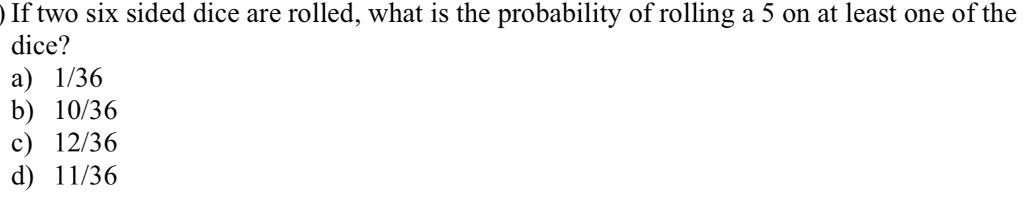For two sided dice, sample space is as below: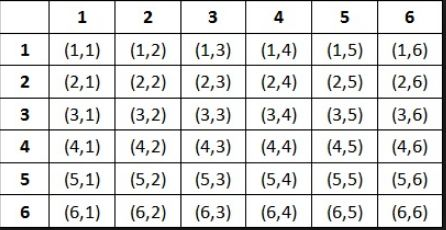Here we see that for 11 times we find rolling on 5 at lease one of the dice.

So the required probability is 11/36

So answer is d. 11/36

#### Earn Coins

Coins can be redeemed for fabulous gifts.

Similar Homework Help Questions
• ### A single six sided dice is rolled. Find the probability of rolling a seven

A single six sided dice is rolled. Find the probability of rolling a seven

• ### A standard pair of six-sided dice is rolled. What is the probability of rolling a sum...

A standard pair of six-sided dice is rolled. What is the probability of rolling a sum less than 5? Express your answer as a fraction or a decimal number rounded to four decimal places.

• ### A standard pair of six-sided dice is rolled. What is the probability of rolling a sum...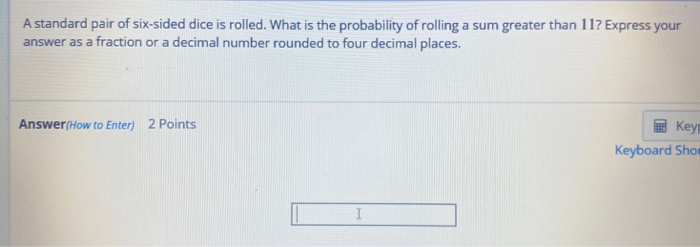A standard pair of six-sided dice is rolled. What is the probability of rolling a sum greater than 117 Express your answer as a fraction or a decimal number rounded to four decimal places. Answer/How to Enter) 2 Points Key Keyboard Shor I

• ### A standard pair of six-sided dice is rolled. What is the probability of rolling a sum...

A standard pair of six-sided dice is rolled. What is the probability of rolling a sum greater than 9? Express your answer as a fraction or a decimal number rounded to four decimal places.

• ### Two fair six-sided dice are rolled. What is the probability that one die shows exactly three...

Two fair six-sided dice are rolled. What is the probability that one die shows exactly three more than the other die (for example, rolling a 1 and 4, or rolling a 6 and a 3)

• ### Correct A standard pair of six-sided dice is rolled. What is the probability of rolling a...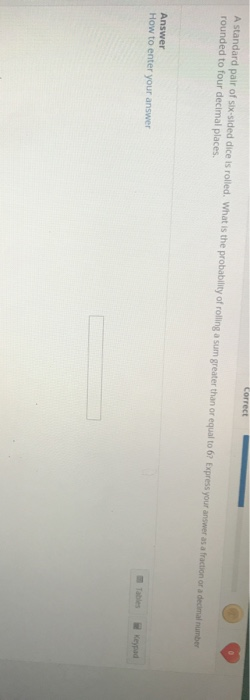Correct A standard pair of six-sided dice is rolled. What is the probability of rolling a sum greater than or equal to 62 Express your answer as a fraction or a decimal number rounded to four decimal places Answer How to enter your answer

• ### Two six-sided dice are rolled. Determine the probability of the following events: a) The second dice...

Two six-sided dice are rolled. Determine the probability of the following events: a) The second dice shows the number two. b) The sum of dice is nine or more. c) None of the dice show a one. I would like a full solution for all of these three questions, if possible please.

• ### If a blue and a red dice are rolled, what is the probability of rolling two identical numbers?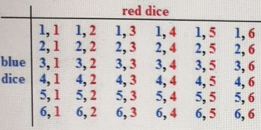If a blue and a red dice are rolled, what is the probability of rolling two identical numbers? A. 18/36 B. 12/36 C. 6/36 D.36/36

• ### Three fair six-sided dice are rolled. a) What is the probability of seeing {1, 3, 6}?...

Three fair six-sided dice are rolled. a) What is the probability of seeing {1, 3, 6}? b) What is the probability of seeing {1, 4, 4}? c) What is the probability of seeing {2, 2, 2} ? d) What is the probability of seeing at least one 6? e) What is the probability that the sum of all three dice is 16? f) What is the probability of seeing exactly two even numbers?

• ### What is the marginal probability of rolling a 2 using two six-sided dice? Round your answer...

What is the marginal probability of rolling a 2 using two six-sided dice? Round your answer to three decimal places What is the probability of rolling something other than double 2s using two six-sided dice? Round your answer to three decimal places